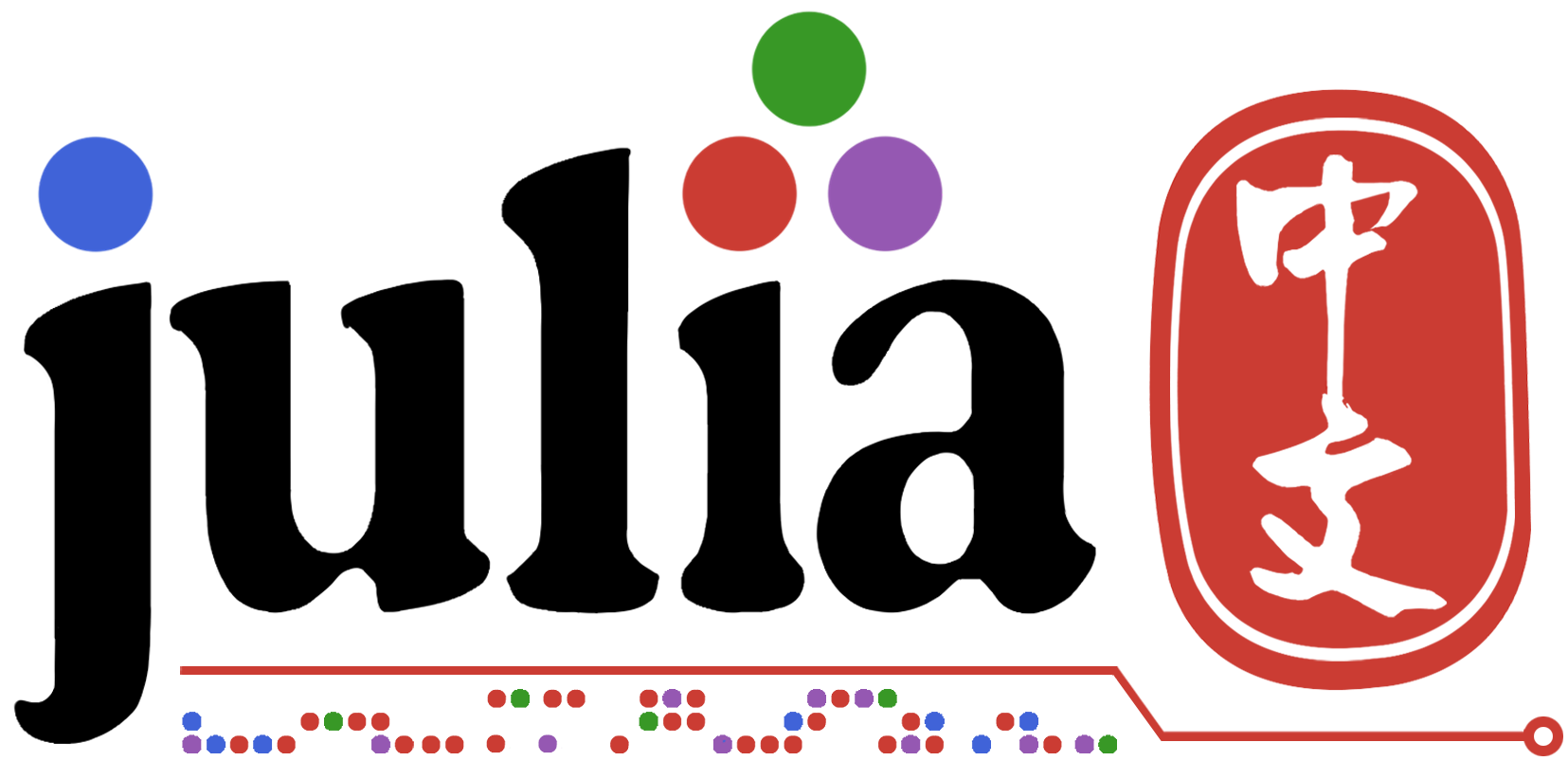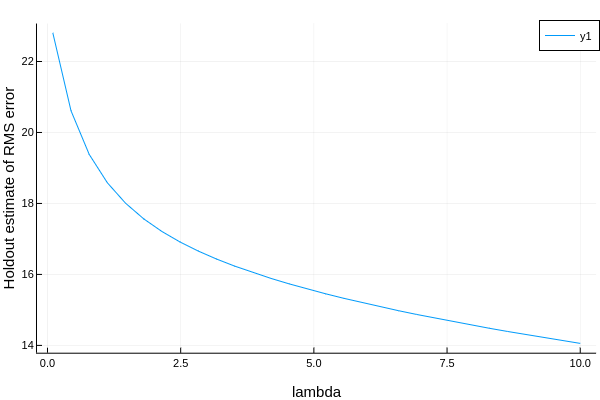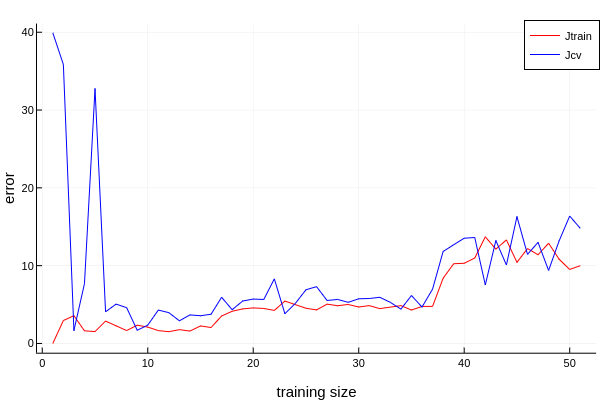# [MLJ]评估模型笔记

## 1. 一个例子

``````X = (a = rand(12), b = rand(12), c = rand(12))
y = X.a .+ 2 .* X.b + 0.05 .* rand(12)
``````

``````model = @load RidgeRegressor pkg=MultivariateStats
``````

[评估]单个measure

``````rng = StableRNG(1234)
cv = CV(nfolds = 3, shuffle = true) # 重采样策略
evaluate(model, X, y, resampling = cv, measure = l2)

# 也可以这样, 下面也是
mach = machine(model, X, y)
evaluate!(mach, resampling = cv, measure = l2)
``````
_.measure _.measurement _.per_fold
l2 0.164 [0.105, 0.23, 0.158]

_.per_observation = [[[0.288, 0.128, …, 0.186], [0.136, 0.534, …, 0.348], [0.435, 0.0345, …, 0.298]], missing, missing]

[评估] 多个measure

``````evaluate(model, X, y, resampling = cv, measure = [l1, rms, rmslp1])
``````
_.measure _.measurement _.per_fold
l1 0.35 [0.505, 0.319, 0.226]
rms 0.424 [0.51, 0.454, 0.273]
rmslp1 0.197 [0.193, 0.256, 0.116]

_.per_observation = [[[0.61, 0.514, …, 0.414], [0.00912, 0.486, …, 0.0136], [0.139, 0.144, …, 0.491]], missing, missing]

``````•   measure: the vector of specified measures

•   measurements: the corresponding measurements, aggregated across the
test folds using the aggregation method defined for each measure
(do aggregation(measure) to inspect)

•   per_fold: a vector of vectors of individual test fold evaluations
(one vector per measure)

•   per_observation: a vector of vectors of individual observation
evaluations of those measures for which
reports_each_observation(measure) is true, which is otherwise
reported missing.
``````

## 2. 评估模型的必要参数

### 2.1 resampling

#### 2.1.1 Holdout

``````holdout = Holdout(; fraction_train=0.7,
shuffle=nothing,
rng=nothing)
``````

#### 2.1.2.CV

``````cv = CV(; nfolds=6,  shuffle=nothing, rng=nothing)
``````

#### 2.1.3 StratifiedCV

``````stratified_cv = StratifiedCV(; nfolds=6,
shuffle=false,
rng=Random.GLOBAL_RNG)
``````

### 2.2 measure

#### 2.2.1 分类指标

1. 混淆矩阵
Ground Truth
Predicted Positive Negative
True TP FN
False FP TN
1. 由混淆矩阵推导出的概率

• 准确率
• 精确率
• 召回率

[补充] FScore为精确率与召回率的调和平均

#### 2.2.2 回归指标

1. l1
`∑|(Yᵢ - h(xᵢ)|`
2. l2
`∑(Yᵢ - h(xᵢ))²`
3. mae
平均绝对误差
`l1(Ŷ,h(xᵢ)) / n`
4. mse
平均平方误差
`l2(Ŷ,h(xᵢ)) / n`
5. rmse
均方根误差
`√(∑(ŷ - y)²`

ps: 不会用latex，函数我都写在w思维导图里了，详细文档看这里

#### 2.2.3 扩展包 LossFunction

TODO LossFunctions (外部包)查询

The LossFunctions.jl package includes “distance loss” functions for Continuous targets, and “marginal loss” functions for Binary targets. While the LossFunctions,jl interface differs from the present one (for, example Binary observations must be +1 or -1), one can safely pass the loss functions defined there to any MLJ algorithm, which re-interprets it under the hood. Note that the “distance losses” in the package apply to deterministic predictions, while the “marginal losses” apply to probabilistic predictions.

github地址:

`LossFunctions`提供了更多的指标，拿文档里的代码举个例子

``````using LossFunctions

X = (x1=rand(5), x2=rand(5));
y = categorical(["y", "y", "y", "n", "y"]);
w = [1, 2, 1, 2, 3];

mach = machine(ConstantClassifier(), X, y);
holdout = Holdout(fraction_train=0.6);
evaluate!(mach,
measure=[ZeroOneLoss(), L1HingeLoss(), L2HingeLoss(), SigmoidLoss()],
resampling=holdout,
operation=predict,
weights=w)
``````
_.measure _.measurements _.per_fold
ZeroOneLoss 0.4 [0.4]
L1HingeLoss 0.8 [0.8]
L2HingeLoss 1.6 [1.6]
SigmoidLoss 0.848 [0.848]

_.per_observation = [[[0.8, 0.0]], [[1.6, 0.0]], [[3.2, 0.0]], [[1.409275324764612, 0.2860870128530822]]]

TODO 学习曲线

TODO ROC

TODO PR

3赞

## 3. 评估模型的图表

### 3.1 学习曲线

``````  curve = learning_curve(mach; resolution=30,
resampling=Holdout(),
repeats=1,
measure=default_measure(machine.model),
rows=nothing,
weights=nothing,
operation=predict,
range=nothing,
acceleration=default_resource(),
acceleration_grid=CPU1(),
rngs=nothing,
rng_name=nothing)
``````

example 观察一个模型的性能

``````X, y = @load_boston

model = RidgeRegressor()
mach = machine(model, X, y)

r_lambda = range(model, :lambda, lower = 0.01, upper = 10.0, scale = :linear)
``````

``````curves = learning_curve(mach,
range = r_lambda,
measure = rms)
plot(curves.parameter_values,
curves.measurements,
xlab = curves.parameter_name,
ylab = "Holdout estimate of RMS error")

````````````using Plots
rng = StableRNG(1234)

curves = learning_curve(mach,
resampling = CV(nfolds = 6, rng = rng),
range = r_lambda,
measure = rms)
plot(curves.parameter_values,
curves.measurements,
xlab = curves.parameter_name,
ylab = "Holdout estimate of RMS error")

``````### 3.2 ROC

``````fprs, tprs, ts = roc_curve(ŷ, y) = roc(ŷ, y)
``````

1. fprs: 假阳性率
2. tprs: 真阳性率
3. ts: thresholds 阈值

example### 3.3 真正的学习曲线

``````function plot_learning_curve(model, X, y)
mach = machine(model, X, y)
training_size_iter = 5:10:length(y)
errors = ones(length(training_size_iter), 2)
rng = StableRNG(1234)

row = 1                     # for iterate
for training_size = training_size_iter
train, cv = partition(1:training_size, 0.7, rng = rng)
fit_only!(mach, rows = train)

m_train = length(train)
Jtrain = (1 / (2 * m_train)) * reduce(+, map(x -> x^2, predict(mach, rows = train) - y[train]))

m_cv = length(cv)
Jcv = (1 / (2 * m_cv)) * reduce(+, map(x -> x^2, predict(mach, rows = cv) - y[cv]))

errors[row, :] = [Jtrain, Jcv]

row += 1
end

plot(errors,
label = ["Jtrain" "Jcv"],
color = [:red :blue],
xlab = "training size",
ylab = "error")

end
``````

``````@load RidgeRegressor pkg=MultivariateStats
model = RidgeRegressor()

# Tuning
rng = StableRNG(1234)
r_lambda = range(model, :lambda, lower = 0.1, upper = 10.0, scale = :linear)
tuning = Grid(resolution = 100, rng = rng)
resampling = CV(nfolds = 6, rng = rng)
self_tuning_model = TunedModel(model = model,
range = r_lambda,
tuning = tuning,
resampling = resampling,
measure = l1)
self_tuning_mach = machine(self_tuning_model, X, y)
fit!(self_tuning_mach, force = true)

best_model = fitted_params(self_tuning_mach).best_model

plot_learning_curve(best_model, X, y)
``````